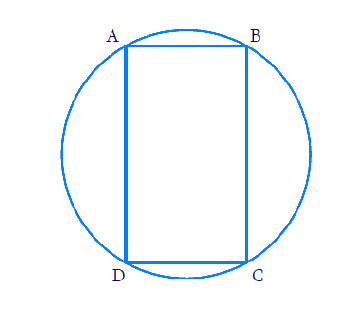In the verge of coronavirus pandemic, we are providing FREE access to our entire Online Curriculum to ensure Learning Doesn't STOP!

# Ex.10.5 Q12 Circles Solution - NCERT Maths Class 9

Go back to  'Ex.10.5'

## Question

Prove that cyclic parallelogram is a rectangle.

Video Solution
Circles
Ex 10.5 | Question 12

## Text Solution

What is given ?

What is unknown?

Prove  that cyclic parallelogram is a rectangle.

Reasoning:

The sum of either pair of opposite angles of a cyclic quadrilateral is $$180^\circ.$$ By using this fact we can show each angle of cyclic parallelogram as $$90^\circ$$ which will prove the statement it is a rectangle.

Steps:Let $${ABCD}$$ be the cyclic parallelogram.

We know that opposite angles of a parallelogram are equal.

\begin {align}\angle {A}=\angle {C} \text { and } \angle {B}=\angle {D} \ldots .(1) \end {align}

We know that the sum of either pair of opposite angles of a cyclic quadrilateral is $$180^{\circ}.$$

\begin {align} \angle {A}+\angle {C}=180^{\circ} \ldots .(2) \end {align}

Substituting ($$1$$) in ($$2$$),

\begin{align}\angle {A}+\angle {C}&=180^{\circ} \\ \angle {A}+\angle {A}&=180^{\circ} \\ 2 \angle {A}&=180^{\circ} \\ \angle {A}&=90^{\circ}\end{align}

We know that if one of the interior angles of a parallelogram is $$90^{\circ},$$ all the other angles will also be equal to $$90^{\circ}.$$

Since all the angles in the parallelogram is $$90^{\circ},$$ we can say that parallelogram $${ABCD}$$ is a rectangle.

Video Solution
Circles
Ex 10.5 | Question 12

Learn from the best math teachers and top your exams

• Live one on one classroom and doubt clearing
• Practice worksheets in and after class for conceptual clarity
• Personalized curriculum to keep up with school